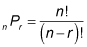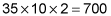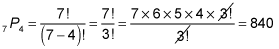##### GED Mathematical Reasoning Test For DummiesThe GED Mathematical Reasoning test may ask you to determine the number of ways two or more items can be arranged or combined. In this case, you're looking at a combinations or permutations problem.

When you look at the supplied formula sheet, you won't find anything about permutations and combinations, so you'll have to memorize the following formula to solve permutations problems:where P stands for permutations, n is the total number of things to choose from, and r is the number chosen.

The following practice questions will get you started with these types of problems.

## Practice questions

1. At a local ice cream store, you can choose from 35 flavors of ice cream, 10 different toppings, and 2 containers — dish or cone. How many different combinations do you have to choose from?

2. How many different 4-letter combinations can be formed from the word STUMPED?

1. 700 ice cream combinations

To answer this question, simply multiply all the numbers:2. 840 possible words

To answer this question, count the number of letters in STUMPED, and you get 7. That's n. You're choosing only 4 letters, so r is 4. Plug the numbers into the permutations equation, and you get your answer: